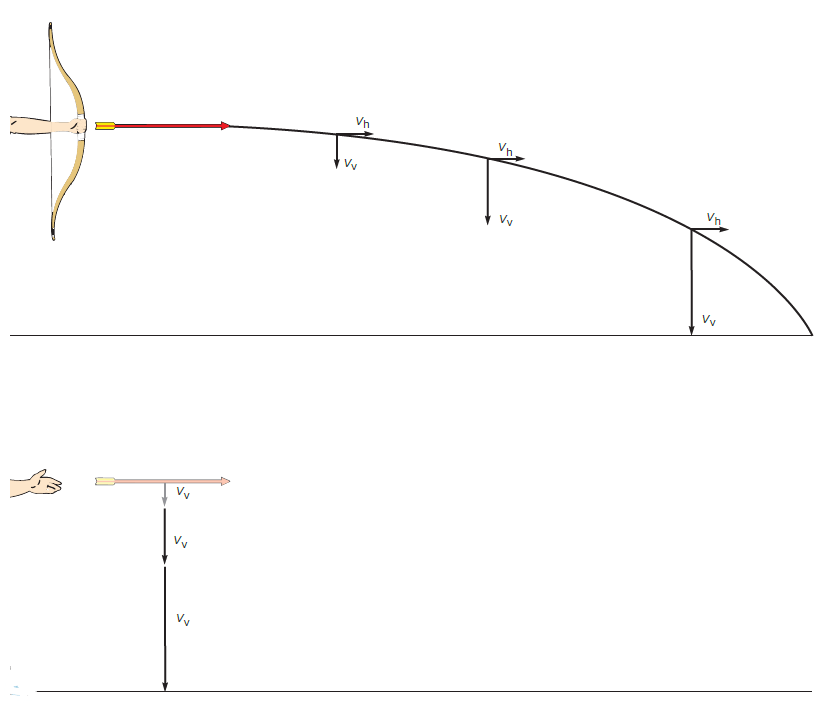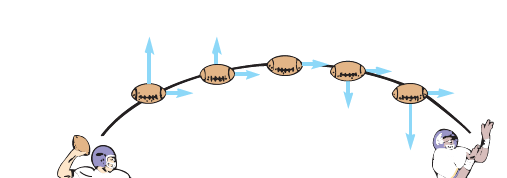# Vertical Projectiles vs. Horizontal Projectiles – a detailed comparison

In this post, we will make a comparison between Vertical projectiles and Horizontal projectiles. When an object is thrown or projected, into the air it’s named a projectile. Essentially, such a projectile (rock, football, bullet, golf ball, or whatever) could be directed straight upward as a vertical projection, directed straight out as a horizontal projection, or directed at some angle between the vertical and the horizontal. For such compound motion, we can observe the following:(1) gravity acts on objects at all times, no matter where they are, and (2) the acceleration due to gravity (g) is independent of any motion that an object may have.

Vertical Projectiles vs. Horizontal Projectiles: In brief, the vertical projectile has only vertical motion, but the horizontal projectile has both vertical motion and horizontal motion. The vertical projectile does not make any horizontal displacement, but the horizontal projectile undergoes a horizontal displacement along with its vertical motion.

Now, we will discuss the features of different types of projectiles.

## Vertical projectiles – features

Consider first a ball that you throw straight upward, a vertical projection. Here, the ball acts as a vertical projectile.
Let’s list down the characteristics of vertical projectiles:

• The ball has an initial velocity but then reaches a maximum height, stops for an instant, then accelerates back toward Earth.
• Gravity is acting on the ball throughout its climb, stop, and fall.
• As it is climbing, the constant force of gravity is continually reducing its velocity.
• The overall effect during the climb is deceleration, which continues to slow the ball until the instantaneous stop.
• After the instantaneous stop at the maximum height, the ball then continues its constant acceleration and moves back to the surface just like a ball that has been dropped from the same height.
• Note that the falling ball has the same speed in the opposite direction that it had on the way up.
• The velocity arrows for a ball thrown straight up are shown in Figure1.

## Horizontal projectiles – features

Horizontal projectiles are easier to understand if you split the complete motion into separate vertical and horizontal parts.
Consider, for example, an arrow shot horizontally from a bow. Here are the features of this horizontal projectile motion.

• The force of gravity accelerates the arrow downward, giving it an increasing downward velocity as it moves through the air. This increasing downward velocity is shown in Figure 2 as increasingly longer velocity arrows (Vv).
• There are no forces in the horizontal direction if you ignore air resistance, so the horizontal velocity of the arrow remains the same throughout the flight, as shown by the identically sized Vh velocity arrows.
• The combination of the increasing vertical (Vv) motion and the unchanging horizontal (Vh) motion causes the arrow to follow a curved path until it hits the ground.
• Neglecting air resistance, an arrow shot horizontally(figure 2 – first image) will strike the ground at the same time as one dropped from the same height above the ground,(figure 2 – second image) as shown in figure 2 by the increasing vertical velocity arrows.figure 2: Motion of horizontal projectileNeglecting air resistance, an arrow shot horizontally(figure 2 – first image from top) willstrike the ground at the same time as one dropped from the same height above the ground, as shown here by the increasing vertical velocity arrows. (figure 2 – second image from top)

An interesting prediction that can be made from the shot arrow analysis is that an arrow shot horizontally from a bow will hit the ground at the same time as a second arrow that is simply dropped from the same height (Figure 2).

### Will a bullet dropped at the same time & the same height as one fired horizontally from a rifle touch the ground at the same time?

The answer is yes; both bullets would hit the ground at the same time. Indeed, without air resistance, all the bullets and arrows should hit the ground at the same time if dropped or shot horizontally from the same height.

## A combination of horizontal & vertical projectile takes a parabolic trajectory

• Golf balls, footballs, and baseballs are usually projected upward at some angle to the horizon.
• The horizontal motion of these projectiles is constant as before because there are no horizontal forces involved.
• The vertical motion is the same as that of a ball projected directly upward.
• The combination of these two motions causes the projectile to follow a curved path called a parabola, as shown in Figure 3. As an example take the path of a ball that has been projected at some angle.
• Note the distance that the ball travels as compared to the angle of projection. An angle of projection of 45° results in the maximum distance of travel if air resistance is ignored and if the launch point and the landing point are at the same elevation.

Read our detailed post on this kind of projectile that undergoes a parabolic path here: Projectiles that make parabolic paths – with derivation, formulas, conceptsfigure 3: A football is thrown at some angle to the horizonwhen it is passed downfield. Neglecting air resistance, the horizontalvelocity is a constant, and the vertical velocity decreases, then it increases,just as in the case of a vertical projectile. The combined motion producesa parabolic path.
Scroll to top
error: physicsTeacher.in